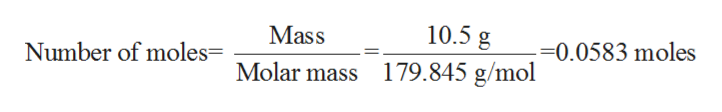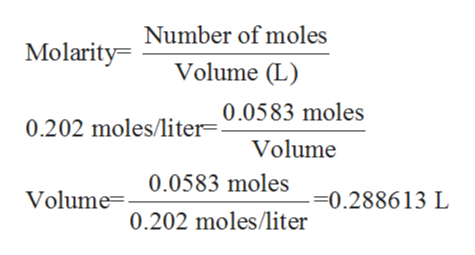How many milliliters of an aqueous solution of 0.202 M iron(II) nitrate is needed to obtain 10.5 grams of the salt?

Question

How many milliliters of an aqueous solution of 0.202 M iron(II) nitrate is needed to obtain 10.5 grams of the salt?

Step 1

The term molarity refers to the ratio of number of moles (ratio of mass to the molar mass) of a substance to the volume of the give n solution in liters. Its SI units are moles/liter, which can also be denoted as ‘M’.

Step 2

In the given problem, the mass of iron (II) nitrate is given to be 10.5 grams while the molarity of the aqueous solution is given to be 0.202 M.

Firstly, the number of moles of iron (II) nitrate are calculated as follows:help_outlineImage Transcriptionclose10.5 g Mass Number of molesMolar mass 179.845 g/mol --0.0583 moles fullscreen
Step 3

Now, the volume can be cal...help_outlineImage TranscriptioncloseMolarity Number of moles Volume (L) 0.202 moles/liter=0.0583 moles Volume 0.0583 moles Volume0.202 moles/liter -0.288613 L fullscreen

Want to see the full answer?

See Solution

Want to see this answer and more?

Our solutions are written by experts, many with advanced degrees, and available 24/7

See Solution
Tagged in

Chemistry